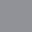It was developed in a secret lab and released, after which it spread rapidly. COVID? (maybe …). But I’m talking about the new PyFL interpreter. It’s finally available for the general public at

pyflang.com

To make things simple, in the form of a zip file   –  I’ll put it  on GitHub if there’s enough interest.

Just read README.txt and follow the instructions. As it says you need Python 3, 3.10.1 being the latest  stable version. . It all should work straight out of the box.

A quick summary of PyFL

It”s all implemented on top of Basic PyFL, a  stripped down lazy functional language. Basic PyFL has the data  types and infix operators of POP-11, lambda expressions, and valof clauses (basically where clauses), and function application. Function application uses conventional mathematical notation, e.g. f(a,b) instead of (f a b) as in Haskell.

Basic PyFL was implemented from scratch using function closures and similar standard techniques. It is  dynamically typed –  no type declarations and no constraints on application (self application in particular              is  supported).

Once Basic PyFL was implemented I proceeded to add more and more extensions, some quite novel.

Conventional function definitions

Mathematicians don’t normally use lambda expressions to define functions. Instead of writing

sumsq = lambda (x,y) x*x+y*y;

they are more likely to write

sumsq(x,y) = x*x+y*y;

This notation is implemented by simple translation – definitions using conventional notation are replaced by the version using lambda. Naturally this involves a complete ‘tree walk’ of the entire program because conventional definitions may occur in values deeply nested in other expressions.

The next feature added is where clauses. These are stylistically more convenient but cause parsing problems and so are not a basic feature. They, too, are implemented by a simple translation. A where clause with subject (head) s and set body of definitions is rewritten as a valof with the same body except that the definition result=s is  added.

With where and lambda we can write a non recursive factorial  program:

fac(7)
where
fac = Y(A);
A(f) = lambda (n) if n==0 then 1 else n*f(n-1) fi;
Y(a) = g(g) where g(x) = a(x(x));
end

Notice that no variable is defined directly or indirectly in terms of itself. This definition of the fixed point combinator Y  clearly depends on self-application, which  Haskell’s  type system won’t allow. I was pretty happy when the PyFL interpreter produced 5040, the  correct answer.

Another feature added was multiple returns, so that you can write e.g.

min, max = bounds(x)

This is implemented with a simple translation but I won’t go in to it.

The next feature added was VBOs – variable binding operators, like the existential quantifier ∃ or the sigma (summation) operator ∑. They work exactly like their math counterparts except the ranges are lists and they are expressed in ASCII  notation. Thus we can compute  the primes less than 100   a

res = [2 3 5 7] <>
those n in rg(2,100):
forall p in [2 3 5 7] :
n mod p != 0
end
end
where
rg(l,u) =
if l>u then []
else l :: rg(l+1,u)
fi;
end;

These VBOs are disguised loops, even though PyFL is a  functional language. They are implemented by translating into expressions using map, filter, and reduce.

My next step was to jump right in and add genuine while loops. We can use a while clause to compute the primes less than 100 as follows

res =
while n <100
n = 3 fby n+2;
nprime = not exist p in primes: n mod p == 0 end;
primes =  fby if nprime then n :: primes else primes fi;
result = primes;
end

2 thoughts on “PyFl – new functional programming language from Bill Wadge”

1.Is PyFL pronounced 'piffle'?

2.